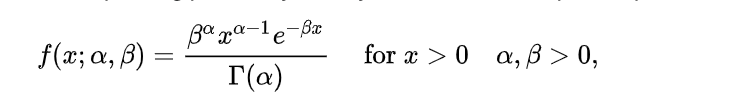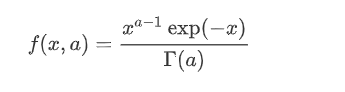# #StackBounty: #bayesian #python #poisson-distribution #gamma-distribution #conjugate-prior How to use scipy stats gamma pdf to update t…

### Bounty: 50

I’m trying to "get my bearings" performing bayesian analysis, specifically I’m exploring the Gamma-Poisson conjugate prior. The definition of the PDF is belowIf the prior takes the form of `gamma(x,a,b)` it’s my understanding that the posterior takes the form `gamma(a,x+n,b+1)` where n is total events in 1 unit of time or `gamma(x, a+<events>, b+<time>)` for multiple units of time.

The issue I’m running into is that scipy (A) defines the Gamma PDF slightly differently, omitting b and is unclear on what the optional variables do, such as `loc` and `scale` (see gamma.pdf.) Consider the scipy definition of the PDF below.My code isn’t working the way that I believe it should.

``````
## class definition
class Gamma():

def __init__(self):
self.a = 1
self.b = 1

def update(self,batch):
time = len(batch) # total units of time
events = sum(batch) # total events across time
self.a += events
self.b += time

def plot(self):
span = np.linspace(0,10,200)
density = gamma.pdf(x=span,a=self.a,scale=self.b)
plt.plot(span,density)

## data generation
data = gamma.rvs(a=3,scale = 1,size = 1000)

## experiment
## Divides data into equally sized batches and updates posterior distribution in each iteration.
g = Gamma()

def experiment_1(var,data,prop=0.2):
size = len(data)
window = int(size*prop)
batches = [batch for batch in range(0,size,window)]
for i in range(1,window):
try:
var.plot()
curr = batches[i]
prev = batches[i-1]
batch = data[prev:curr]
var.update(batch)
except:
pass

experiment_1(var=g,data=data)
``````

Have a look at the plot, which shows the progress through multiple iterations/batches, updating the posterior.The best I can determine is that the scipy.stats.gamma.pdf function’s scale parameter does not function the way that b ought to in the mathematical expression.

What should I do to alter this code such the posterior correctly updates in each iteration?

Edit: I posted a similar question where I did not use scipy for the Gamma distribution, I only used it for the gamma function in the denominator. What I found was that for rates above ~5, the denominator exploded, resulting in numeric overflow and my code crashed.

For my purposes, I need to be plotting events on the scale of about 100-500 per day. I currently have no working solution. Please help me revise code to achieve this desired effect.

Get this bounty!!!

This site uses Akismet to reduce spam. Learn how your comment data is processed.